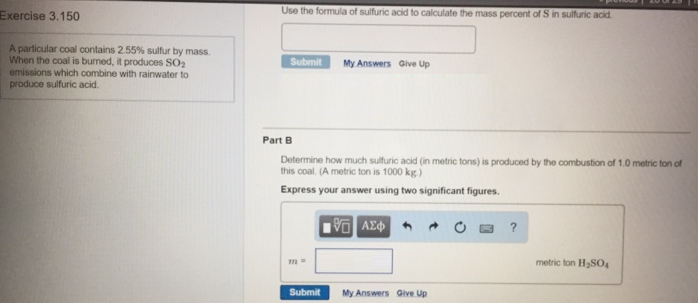# Problem: A particular coal contains 2.55% sulfur by mass. When the coal is burned, it produces SO2 emissions which combine with rainwater to produce sulfuric acid.a. Use the formula of sulfuric acid to calculate the mass percent of S in sulfuric acid.b. Determine how much sulfturic acid (in metric tons) is produced by the combustion of 1.0 metric ton of this coal. (A metric ton is 1000 kg.)

###### FREE Expert Solution
81% (476 ratings)###### Problem Details

A particular coal contains 2.55% sulfur by mass. When the coal is burned, it produces SO2 emissions which combine with rainwater to produce sulfuric acid.

a. Use the formula of sulfuric acid to calculate the mass percent of S in sulfuric acid.

b. Determine how much sulfturic acid (in metric tons) is produced by the combustion of 1.0 metric ton of this coal. (A metric ton is 1000 kg.)